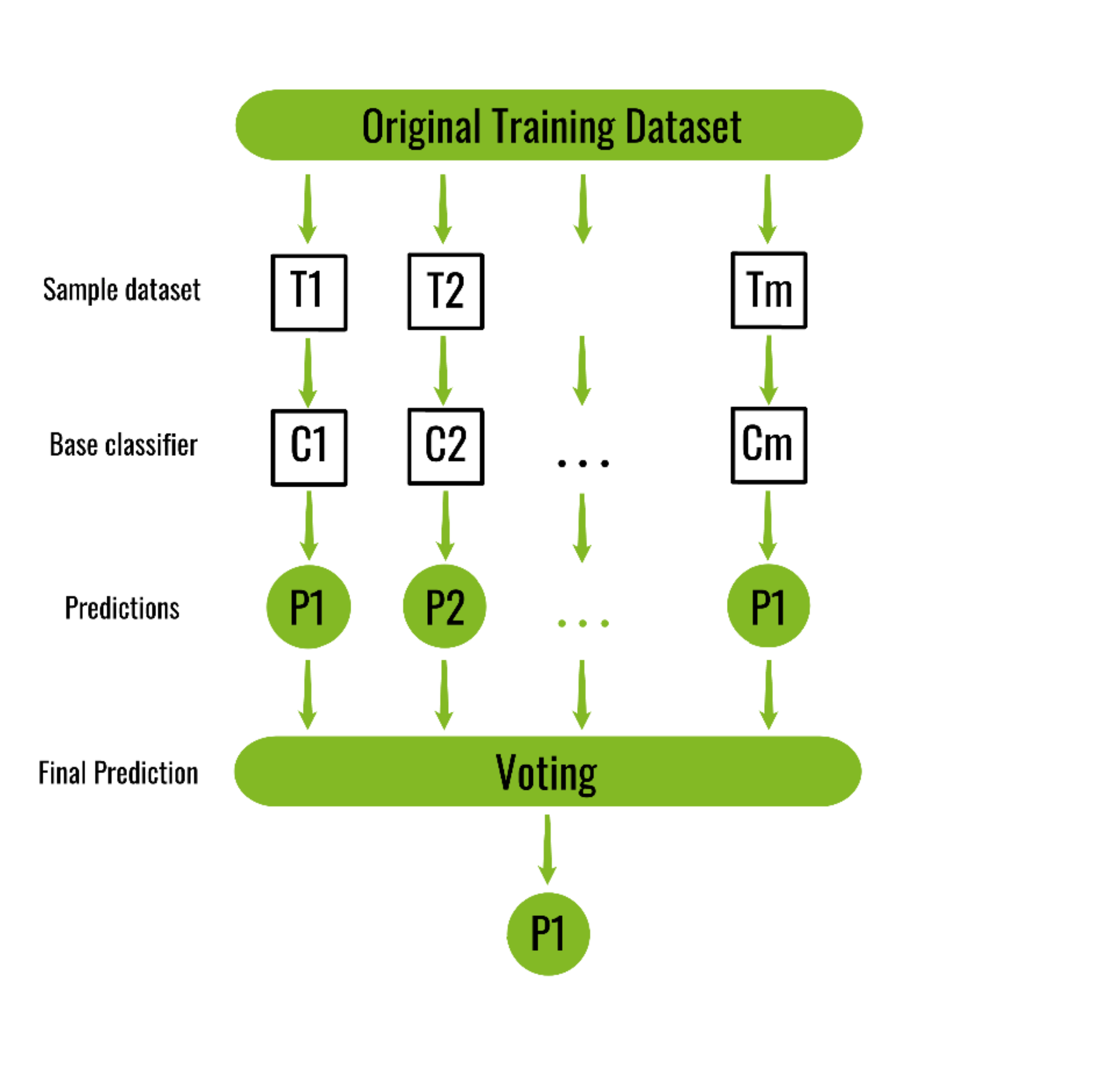Open in App
Not now

# ML | Bagging classifier

• Last Updated : 23 Jan, 2023

A Bagging classifier is an ensemble meta-estimator that fits base classifiers each on random subsets of the original dataset and then aggregates their individual predictions (either by voting or by averaging) to form a final prediction. Such a meta-estimator can typically be used as a way to reduce the variance of a black-box estimator (e.g., a decision tree), by introducing randomization into its construction procedure and then making an ensemble out of it. Each base classifier is trained in parallel with a training set which is generated by randomly drawing, with replacement, N examples(or data) from the original training dataset – where N is the size of the original training set. The training set for each of the base classifiers is independent of each other. Many of the original data may be repeated in the resulting training set while others may be left out. Bagging reduces overfitting (variance) by averaging or voting, however, this leads to an increase in bias, which is compensated by the reduction in variance though.

How does Bagging work on the training datasets?

How bagging works on an imaginary training dataset is shown below. Since Bagging resamples the original training dataset with replacement, some instance(or data) may be present multiple times while others are left out.

Bagging, which stands for Bootstrap Aggregating, is an ensemble machine learning technique that combines the predictions of multiple models to improve the overall performance of the system. The Bagging Classifier is an ensemble method that uses bootstrap resampling to generate multiple different subsets of the training data, and then trains a separate model on each subset. The final predictions are made by combining the predictions of all the models.

One of the main advantages of Bagging is that it can reduce the variance of the model, by averaging the predictions of multiple models. It can also help to reduce overfitting, as the models are trained on different subsets of the data, which can help to reduce the correlation between the models.

The Bagging classifier is a general-purpose ensemble method that can be used with a variety of different base models, such as decision trees, neural networks, and linear models. It is also an easy-to-use and effective method for improving the performance of a single model.

The Bagging classifier can be used to improve the performance of any base classifier that has high variance, for example, decision tree classifiers. The Bagging classifier can be used in the same way as the base classifier with the only difference being the number of estimators and the bootstrap parameter.

In summary, Bagging is an ensemble technique that uses bootstrap resampling to generate multiple different subsets of the training data, and then trains a separate model on each subset. The Bagging Classifier can be used to improve the performance of any base classifier that has high variance, it reduces the variance of the model and can help to reduce overfitting. The Bagging classifier is a general-purpose ensemble method that can be used with a variety of different base models.

Original training dataset: 1, 2, 3, 4, 5, 6, 7, 8, 9, 10 Resampled training set 1: 2, 3, 3, 5, 6, 1, 8, 10, 9, 1 Resampled training set 2: 1, 1, 5, 6, 3, 8, 9, 10, 2, 7 Resampled training set 3: 1, 5, 8, 9, 2, 10, 9, 7, 5, 4

Algorithm for the Bagging classifier:

```Classifier generation:

Let N be the size of the training set.
for each of t iterations:
sample N instances with replacement from the original training set.
apply the learning algorithm to the sample.
store the resulting classifier.

Classification:
for each of the t classifiers:
predict class of instance using classifier.
return class that was predicted most often.```Below is the Python implementation of the above algorithm:

## Python3

 `from` `sklearn ``import` `model_selection` `from` `sklearn.ensemble ``import` `BaggingClassifier` `from` `sklearn.tree ``import` `DecisionTreeClassifier` `import` `pandas as pd`   `# load the data` `url ``=` `"``/``home``/``debomit``/``Downloads``/``wine_data.xlsx"` `dataframe ``=` `pd.read_excel(url)` `arr ``=` `dataframe.values` `X ``=` `arr[:, ``1``:``14``]` `Y ``=` `arr[:, ``0``]`   `seed ``=` `8` `kfold ``=` `model_selection.KFold(n_splits ``=` `3``,` `                       ``random_state ``=` `seed)`   `# initialize the base classifier` `base_cls ``=` `DecisionTreeClassifier()`   `# no. of base classifier` `num_trees ``=` `500`   `# bagging classifier` `model ``=` `BaggingClassifier(base_estimator ``=` `base_cls,` `                          ``n_estimators ``=` `num_trees,` `                          ``random_state ``=` `seed)`   `results ``=` `model_selection.cross_val_score(model, X, Y, cv ``=` `kfold)` `print``("accuracy :")` `print``(results.mean())`

Output:

```accuracy :
0.8372093023255814```

My Personal Notes arrow_drop_up
Related Articles